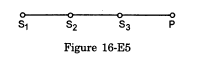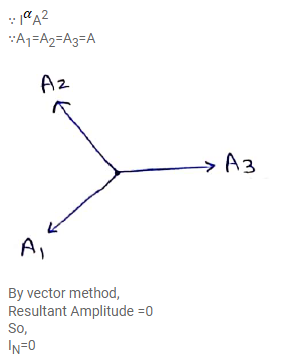# Three sources of sound S1, S2 and S3 of equal intensity are placedQuestion:

Three sources of sound $S_{1}, S_{2}$ and $S_{3}$ of equal intensity are placed in a straight line with $S_{1} S_{2}=S_{2} S_{3}$. At a point $P_{1}$ far away from the sources, the wave coming from $S_{2}$ is $120^{\circ}$ ahead in phase of that from $S_{1}$. Also, the wave coming from $S_{3}$ is $120^{\circ}$ ahead of that from $S_{2}$. What would be the resultant intensity of sound at $P$ ?Solution: